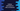# Python program to convert kilometers to miles## Convert Kilometer :

In this python programming tutorial, we will learn how to convert a kilometer value to miles. The program will take the kilometer input from the user, convert it and print out the result.

## Algorithm :

The following algorithm we will use :

1. Ask the user to enter the kilometer value.
2. 1 kilometer is approx 0.621371 miles. So, multiply the user input value with 0.621371 to convert it to miles.
3. Print out the result to the user.
4. Exit the program.

## Python program :

``````fact = 0.621371

km = float(input("Enter the kilometer value : "))
miles = km * fact

print("{} km = {} miles".format(km,miles))``````

### Explanation :

As you can see, we are reading the kilometer value as float _and calculated the value of the miles by multiplying it with _fact _or _0.621371. Finally, we are printing the result.

### Sample Output :

``````Enter the kilometer value : 3.5
3.5 km = 2.1747985 miles

Enter the kilometer value : 2
2.0 km = 1.242742 miles

Enter the kilometer value : 10
10.0 km = 6.21371 miles

Enter the kilometer value : 100
100.0 km = 62.137100000000004 miles

Enter the kilometer value : 40
40.0 km = 24.85484 miles``````

### Conclusion :

In this tutorial, we have learned how to convert kilometer value to mile. You can also change this program to convert a mile value to a kilometer. Try to run the program and drop one comment below if you have any queries.